# Schottky Effect

Points to understand which are related to Schottky effect are the following:
In a solid material, there will be two or less than two electrons for each atom which are free to move from one atom to another based on band theory.

Work Function: The bare minimum quantity of energy (produced due to thermal energy) essential for an electron to escape (leave) from the surface of a material is called work function.

Thermionic Emission: It is the emission (release) of charge carriers (ions or electrons) from the surface of a material due to the thermal energy given to it.

Thermionic Emission Current: As a result of thermionic emission (electron ejection due to thermal energy), an electric current will be produced across the electrodes which is termed as thermionic emission current.

Work function is inversely proportional to thermionic emission current.

Richardson’s Law: It is mathematically analogous to Arrhenius equation. It gives the relationship between the current and the temperature of a heated wire. That is, the current will depend on the temperature of the wire exponentially as shown below.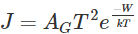J → Emission Current density
W → Work function of the metal
T → Temperature of the metal
K → Boltzmann constant =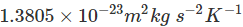AG = λR A0
λR → Material specific correction factor (typically of order 0.5)
A0 → Universal constant =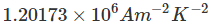Now, we can come to the topic – Schottky Effect (Field enhanced thermionic emission). Here; when we apply an electric field to a heated material, the emission of electrons from the surface increases. It results in decreasing the smallest energy required for the release of electrons from the surface of the material (work function) and thereby increasing thermionic current. If a very small electric field is applied, then it only sweeps the previously released electrons from the surface of the material. While increasing the field (moderate field), a particular point is reached at which the work function value gets decreased. If the field is further increased, it results in decreasing in work function and thereby increasing the thermionic emission current. This is Schottky effect.

In the absence of electric field, the surface barrier which is seen by the emitting Fermi-level electron will be W height. This height is equal to the work function. But the electric field will decrease the barrier height by an amount equal to ΔW (see figure 2) and thus increasing the thermionic current. This can be modelled by an easy alteration in Richardson equation which is explained before. In that equation, we can substitute W by W + ΔW as shown below.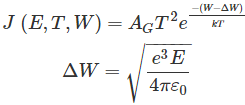The above equation satisfies only for electric field strength less than 108 V/m. But for very high increase in electric field; it results in tunnelling of electrons (excessive electron emission) through the potential barrier. This type of emission or tunnelling is called as high-field emission or simply we can say field emission.
When we plot a graph of logarithm of thermionic emission current and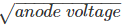will give a straight line (shown below). At low anode voltage, the deviation is due to space charge effects.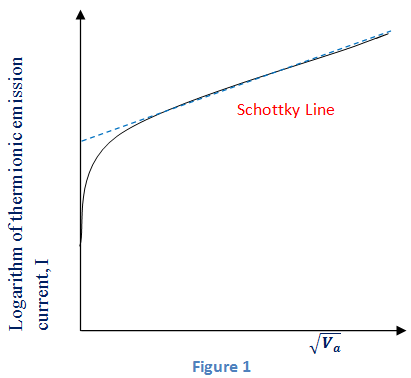The electric lines of force are at 90o to the surface of a metal due to high conductivity (see figure below). The inter-atomic distance is assumed to be ‘a’. Thus, an electron which is located at a distance x > a from the surface having charge – e interacts with surface. It induces an electrical image (+e) in the metal which will be at a distance of x. There will be an attractive force between – e and + e given by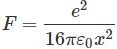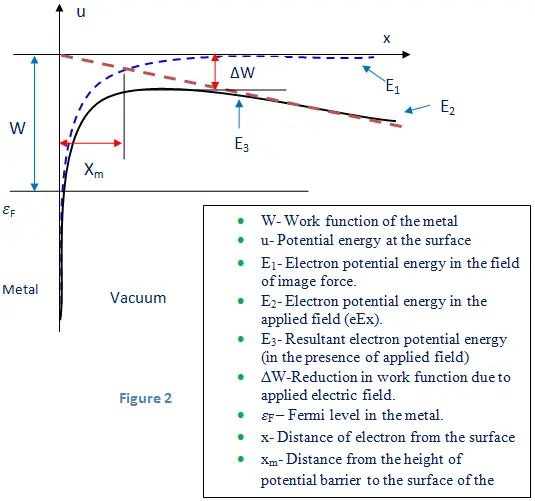Want To Learn Faster? 🎓
Get electrical articles delivered to your inbox every week.
No credit card required—it’s 100% free.